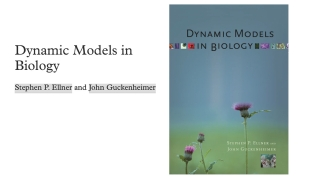# What Are Dynamic Models? Chapter 1 from Dynamic Models in BiologyBeginner
Book
Theory

Throughout this book we use a wide-ranging set of case studies to illustrate different aspects of models and modeling. In this introductory chapter we describe and give examples of different types of models and their uses. We begin by describing the principles that we use to formulate dynamic models, and then give examples that illustrate the range of model types and applications. We do only a little bit of mathematics, some algebra showing how a model for enzyme kinetics can be replaced by a much simpler model which can be solved explicitly. We close with a general discussion of the final “dimension” listed above—the different purposes that dynamic models serve in biology. This chapter is intended to provide a basic conceptual framework for the study of dynamic models—what are they? how do they differ from other kinds of models? where do they come from?—and to flesh out the framework with some specific examples. Subsequent chapters are organized around important types of dynamic models that provide essential analytical tools, and examples of significant applications that use these methods. We explain just enough of the mathematics to understand and interpret how it is used to study the models, relying upon computers to do the heavy lifting of mathematical computations.

This resource is included in the following topics and journeys: